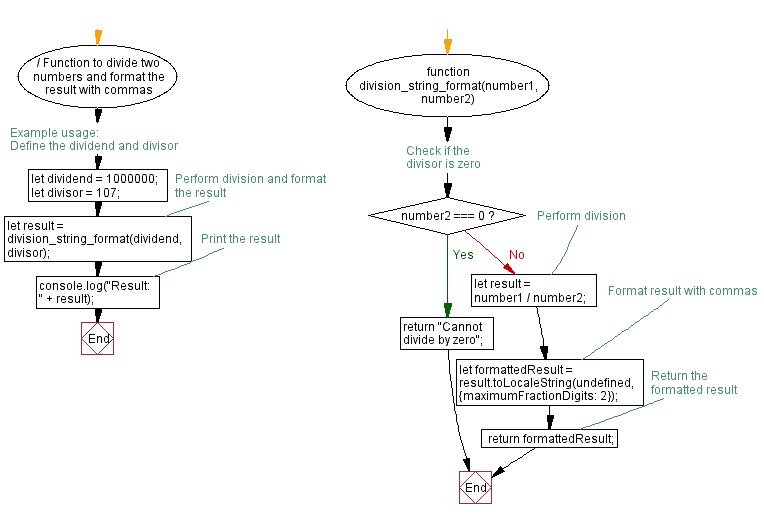# JavaScript: Divide two positive numbers and return a string with properly formatted commas

## JavaScript Basic: Exercise-56 with Solution

Write a JavaScript program to divide two positive numbers and return a string with properly formatted commas.

Sample Solution:

HTML Code:

``````<!DOCTYPE html>
<html>
<meta charset="utf-8">
<meta name="viewport" content="width=device-width">
<title>JavaScript program to divide two positive numbers and return a string with properly formatted commas.</title>
<body>

</body>
</html>
```
```

JavaScript Code:

``````function division_string(n1, n2) {

n1 = 80;
n2 = 6;

var div = Math.round(n1 / n2).toString(),
result_array = div.split("");

if (div >= 1000)
{
for (var i = div.length - 3; i > 0; i -= 3)
{
result_array.splice(i, 0, ",");
}
result_array;
}
console.log(result_array);
```
```

Sample Output:

```["1","3"]
```

Flowchart:Live Demo:

See the Pen JavaScript - Check if a given string contains equal number of p's and t's present - basic-ex-55 by w3resource (@w3resource) on CodePen.

What is the difficulty level of this exercise?

Test your Programming skills with w3resource's quiz.

﻿

## JavaScript: Tips of the Day

Converts a string to title case

Example:

```const toTitleCase = str =>
str
.match(/[A-Z]{2,}(?=[A-Z][a-z]+[0-9]*|\b)|[A-Z]?[a-z]+[0-9]*|[A-Z]|[0-9]+/g)
.map(x => x.charAt(0).toUpperCase() + x.slice(1))
.join(' ');
console.log(toTitleCase('some_database_field_name')); // 'Some Database Field Name'
console.log(toTitleCase('Some label that needs to be title-cased')); // 'Some Label That Needs To Be Title Cased'
console.log(toTitleCase('some-package-name')); // 'Some Package Name'
console.log(toTitleCase('some-mixed_string with spaces_underscores-and-hyphens')); // 'Some Mixed String With Spaces Underscores And Hyphens'
```

Output:

```"Some Database Field Name"
"Some Label That Needs To Be Title Cased"
"Some Package Name"
"Some Mixed String With Spaces Underscores And Hyphens"
```